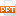# Constraint satisfaction problems

Constraint programming is the study of computational systems based on constraints. The idea of constraint programming is to solve problems by stating constraints about the problem area and, consequently, finding solution satisfying all the constraints. As for the expressiveness of the language, Constraint Programming provides the user to manipulate directly: computational domains much closer to the user’s domain (e.g. natural numbers, rational terms, boolean formulas) different types of numerical and symbolic constraint (e.g. equality, disequality, inequalities, functional) As for the efficiency, Constraint Programming systems use more mathematical techniques, namely constraint solving techniques.55 trang | Chia sẻ: dntpro1256 | Lượt xem: 477 | Lượt tải: 0
Bạn đang xem trước 20 trang tài liệu Constraint satisfaction problems, để xem tài liệu hoàn chỉnh bạn click vào nút DOWNLOAD ở trên
•csp_4271_1810890.ppt